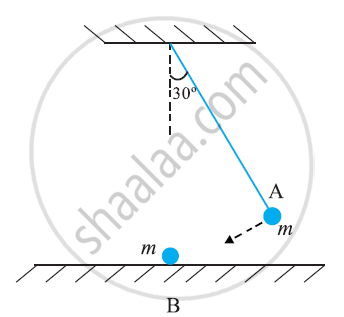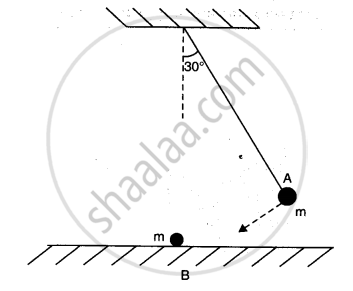CBSE (Science) Class 11CBSE
Share

# The Bob a of a Pendulum Released from 30° to the Vertical Hits Another Bob B of the Same Mass at Rest on a Table as Shown in Fig. How High Does the Bob a Rise After the Collision? Neglect the Size of the Bobs and Assume the Collision to Be Elastic - CBSE (Science) Class 11 - Physics

ConceptConcept of Collisions

#### Question

The bob A of a pendulum released from 30° to the vertical hits another bob B of the same mass at rest on a table as shown in Fig. How high does the bob A rise after the collision? Neglect the size of the bobs and assume the collision to be elastic.#### Solution 1

The bob A will not rise because when two bodies of same mass undergo an elastic collision, their velocities are interchanged. After collision, ball A will come to rest and the ball B would move with the velocity of A, Figure. Thus the bob A will not rise after the collision.

#### Solution 2

Since collision is elastic therefore A would come to rest and B would begin to move with the velocity of A.The bob transfers its entire momentum to the  ball on the table. The bob does not rise at all.

Is there an error in this question or solution?

#### APPEARS IN

NCERT Solution for Physics Textbook for Class 11 (2018 to Current)
Chapter 6: Work, Energy and Power
Q: 17 | Page no. 137
Solution The Bob a of a Pendulum Released from 30° to the Vertical Hits Another Bob B of the Same Mass at Rest on a Table as Shown in Fig. How High Does the Bob a Rise After the Collision? Neglect the Size of the Bobs and Assume the Collision to Be Elastic Concept: Concept of Collisions.
S